# Pythagorean Theorem

### a²  +  b²  =  c²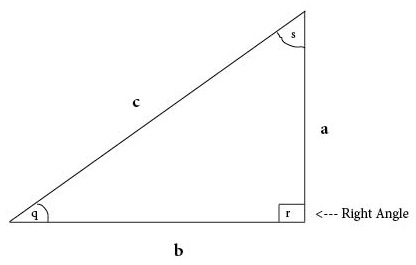Basics
Pythagorean Theorem:  a² + b² = c²
a = altitude = height
b = base
c = hypotenuse

The Pythagorean Theorem applies to a right triangle (a triangle in which one of the angles measures 90°).

Questions involving the Pythagorean Theorem require the calculation of squares and square roots.is the squaring command.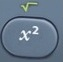is the square root command.activates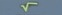above.

Formulas (provided by Mathematics Formula Sheet)

a² + b² = c²

Formulas (NOT provided by Mathematics Formula Sheet)

c² = a² + b²
c = √

b² = c² – a²
b = √

a² = c² – b²
a = √

Question
In a right triangle, a = 5 and b = 12.  What is c?

c = 13

c² = a² + b²
c² = 5² + 12²
c² = 25 + 144
c² = 169

c = √
c = √169
c = 13

Calculator Click What You See Commentblinker clears screen+ 125²+12² c² = a² + b²169169 169 c = √13 Answer
###### Pythagorean Theorem

Question
In a right triangle, a = 6 and c = 10.  What is b?

b = 8

b² = c² – a²
b² = 10² – 6²
b² = 100 – 36
b² = 64

b = √
b = √64
b = 8

Calculator Click What You See Commentblinker clears screen
10– 610²-6² b² = c² – a²6464 64 b = √8 Answer
###### Pythagorean Theorem

Question
In a right triangle, b = 3 and c = 5.  What is a?

a = 4

a² = c² – b²
a² = 5² – 3²
a² = 25 – 9
a² = 16

a = √
a = √16
a = 4

Calculator Click What You See Commentblinker clears screen– 35²-3² a² = c² – b²1616 16 a = √4 Answer
###### Pythagorean Theorem

Question
A right triangular billboard has a height of 6 feet and base of 8 feet.
How long is its hypotenuse?

10 feet

c² = a² + b²
c² = 6² + 8²
c² = 36 + 64
c² = 100

c = √
c = √100
c = 10

Calculator Click What You See Commentblinker clears screen+ 86²+8² c² = a² + b²100100 100 c = √10 Answer
###### Pythagorean Theorem

Question
A right triangular billboard has a height of 5 feet and hypotenuse of 13 feet.
How long is its base?

12 feet

b² = c² – a²
b² = 13² – 5²
b² = 169 – 25
b² = 144

b = √
b = √144
b = 12

Calculator Click What You See Commentblinker clears screen
13– 513²-5² b² = c² – a²144144 144 b = √12 Answer
###### Pythagorean Theorem

Question
A right triangular billboard has a base of 12 feet and hypotenuse of 20 feet.
How high is it?

16 feet

a² = c² – b²
a² = 20² – 12²
a² = 400 – 144
a² = 256

a = √
a = √256
a = 16

Calculator Click What You See Commentblinker clears screen
20– 1220²-12² a² = c² – b²256256 256 a = √16 Answer
###### Pythagorean Theorem

QuestionA snail can crawl 4 feet along a wall and then turn 90° to the left and crawl 3 feet along another wall.  If the snail took a straight shortcut from the start to the end of these two walls, how far would the snail crawl?

5 feet

c² = a² + b²
c² = 3² + 4²
c² = 9 + 16
c² = 25

c = √
c = √25
c = 5

Calculator Click What You See Commentblinker clears screen+ 43²+4² c² = a² + b²2525 25 c = √5 Answer
###### Pythagorean Theorem

QuestionA snail can crawl 4 feet along a wall and then turn 90° to the left and crawl 3 feet along another wall.  If the snail took a straight shortcut from the start to the end of these two walls, how much shorter was the shortcut than sticking to the walls?

2 feet

c² = a² + b²
c² = 3² + 4²
c² = 9 + 16
c² = 25

c = √
c = √25
c = 5

Subtract shortcut distance from wall distance.
a + b – c = 3 + 4 – 5 = 2

Calculator Click What You See Commentblinker clears screen+ 43²+4² c² = a² + b²2525 25 c = √5 c
3 + 4 – 5 3+4-5 Subtract shortcut from walls.
a + b – c2 Answer
###### Pythagorean Theorem

Practice – Questions
1.  In a right triangle, a = 10 and b = 24.  What is c?

2.  In a right triangle, a = 50 and c = 130.  What is b?

3.  In a right triangle, b = 30 and c = 50.  What is a?

4.A ladder is leaning against a wall.  Its base is 4.4 meters from the wall.  Its top is 3.3 meters up the wall.  How long is the ladder?

5.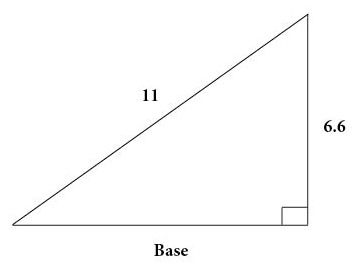A ladder is leaning against a wall.  The ladder is 11 feet long.  Its top is 6.6 feet up the wall.
How long is its base?

6.A ladder is leaning against a wall.  The ladder is 25 feet long.  Its base is 20 feet from the wall.  How high is the ladder up the wall?

7.A snake can slither 32 feet along a wall and then turn 90° to the left and slither 24 feet along another wall.  If the snake took a straight shortcut from the start to the end of these two walls, how far would the snail slither?

8.  With reference to Question 7, how much shorter was the shortcut than sticking to the walls?

9.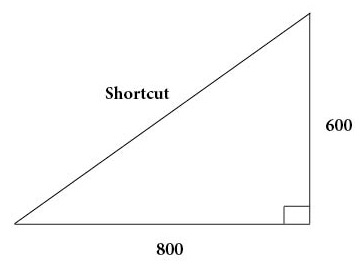A sports fan can run 800 feet along the sideline of a playing field and then turn 90° to the left and run 600 feet along another sideline of a playing field.  At halftime, the sports fan can run a shortcut straight across the playing field from the start to the end of the two sidelines.  What is the distance of this shortcut?

10.  With reference to Question 9, how much longer was sticking to the sidelines than taking the shortcut?

1.  c = 26

2.  b = 120

3.  a = 40

4.  5.5 meters

5.  8.8 feet

6.  15 feet

7.  40 feet

8.  16 feet

9.  1000 feet

10.  400 feet